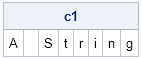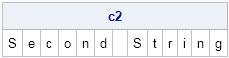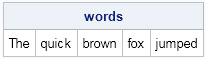Sometimes it is useful in the SAS/IML language to convert a character string into a vector of one-character values. For example, you might want to count the frequency distribution of characters, which is easy when each character is an element of a vector. The question of how to convert a string into a vector was asked recently on the SAS/IML Support Community. I offered two solutions.

In the SAS/IML language, a string of length L is stored as a 1 x 1 matrix. You can use the CSHAPE function to resize character matrices. In particular, you can convert a 1 x 1 matrix with L characters into a 1 x L matrix, where each element of the matrix contains a single character:

```proc iml; start chop(s); return( cshape(s,1,nleng(s),1) ); finish;   c1 = chop( "A String" ); print c1;```If you have SAS/IML 12.1, you can use a new feature of the SUBSTR function to extract substrings of length 1 from the string. In SAS/IML 12.1, the SUBSTR function supports vectors for its position and length arguments, so you can write a single expression that extracts L substrings, each containing one character:

```start chop(s); return ( substr(s, 1:nleng(s), 1) ); /* SAS/IML 12.1 */ finish;   c2 = chop( "Second String" ); print c2;```The new SUBSTR functionality is useful in many other situations. For example, the following call to the SUBSTR function extracts whole words from a space-delimited string. You can use the ANYSPACE function to find the location of blank spaces, and then use the SUBSTR function to extract the words between the spaces. The following example assumes that the location of the spaces and the lengths of the words are already known:

```/* break up a phrase by white space */ s = "The quick brown fox jumped."; pos = {1 5 11 17 21}; lengths = {3 5 5 3 6}; words = substr(s, pos, lengths); print words;```ShareDistinguished Researcher in Computational Statistics

Rick Wicklin, PhD, is a distinguished researcher in computational statistics at SAS and is a principal developer of SAS/IML software. His areas of expertise include computational statistics, simulation, statistical graphics, and modern methods in statistical data analysis. Rick is author of the books Statistical Programming with SAS/IML Software and Simulating Data with SAS.

1.If the objective is to chop a string into words, then there is no need to know the locations and lengths as it can all be done with SCAN. Something like,

words = scan(s, 1:( 1 + countc(s, ' ')));

should do the trick.

•Thanks. The objective was to give another example of using the SUBSTR function, but I like your example. To be more robust, you can include a third argument to the SCAN function so that hyphenated words will be handled correctly.

•Ian Wakeling on

How about using the new substr feature to visualize experimental designs as you might find them printed in books?
.
•